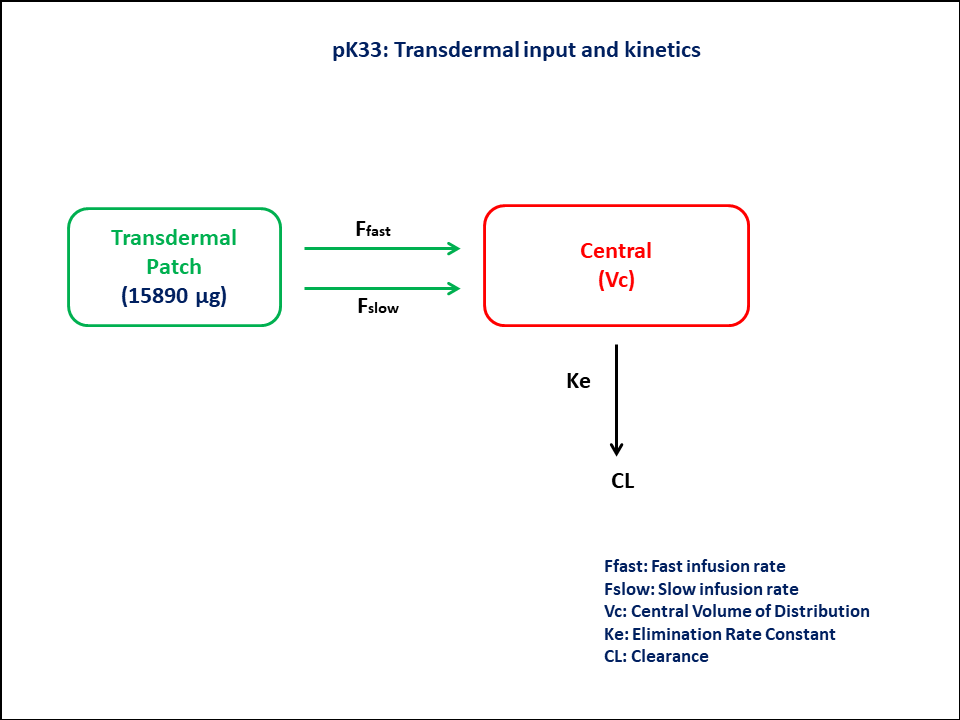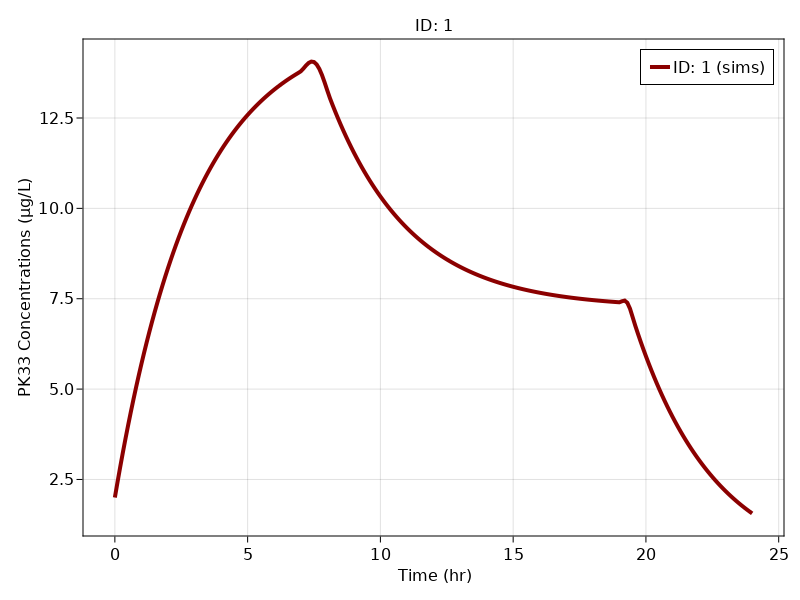# Exercise 33 - Transdermal input and kinetics

### Background

• Structural model - One compartment linear elimination with zero-order input

• Route of administration - Transdermal

• Dosage Regimen - 15,890 μg per patch. The patch was applied for 16 hours for 5 consecutive days

• Number of Subjects - 1### Learning Outcome

To understand the kinetics of a given drug using Transdermal input following 2 different input rates

### Objectives

To build one compartment model with zero-order input and to understand its function using transdermal delivery system.

### Libraries

Call the "necessary" libraries to get started.

using Random
using Pumas
using PumasUtilities
using CairoMakie


### Model

To build one compartment model with zero-order input following transdermal drug administration

pk_33         = @model begin
desc      = "One Compartment Model"
timeu     = u"hr"
end

@param begin
"Clearance (L/hr)"
tvcl      ∈ RealDomain(lower=0)
"Volume of Central Compartment (L)"
tvvc      ∈ RealDomain(lower=0)
"Dose of slow infusion (μg)"
tvdslow   ∈ RealDomain(lower=0)
"Duration of fast release (hr)"
tvtfast   ∈ RealDomain(lower=0)
"Duration of slow release (hr)"
tvtslow   ∈ RealDomain(lower=0)
Ω         ∈ PDiagDomain(5)
"Proportional RUV"
σ²_prop   ∈ RealDomain(lower=0)
end

@random begin
η         ~ MvNormal(Ω)
end

@pre begin
Cl        = tvcl * exp(η)
Vc        = tvvc * exp(η)
Dose_slow = tvdslow * exp(η)
Tfast     = tvtfast * exp(η)
Tslow     = tvtslow * exp(η)
Ffast     = (t<=Tfast) * (15890-Dose_slow)/Tfast
Fslow     = (t<=Tslow) * Dose_slow/Tslow
end

@init begin
Central  = 2*Vc
end

@dynamics begin
Central'  =  Ffast + Fslow -(Cl/Vc)*Central
end

@derived begin
cp        = @. Central/Vc
"""
Observed Concentration (ug/L)
"""
dv        ~ @. Normal(cp, sqrt((cp^2*σ²_prop) + σ_add^2))
end
end

PumasModel
Parameters: tvcl, tvvc, tvdslow, tvtfast, tvtslow, Ω, σ²_prop, σ_add
Random effects: η
Covariates:
Dynamical variables: Central
Derived: cp, dv
Observed: cp, dv


### Parameters

Parameters provided for simulation are as below. tv represents the typical value for parameters.

• $CL$ - Clearance (L/hr),

• $Vc$ - Volume of Central Compartment (L)

• $Dslow$ - Dose of slow infusion (μg)

• $Tfast$ - Duration of fast release (hr)

• $Tslow$ - Duration of slow release (hr)

• $Ω$ - Between Subject Variability

• $σ$ - Residual error

param = ( tvcl    = 79.8725,
tvvc    = 239.94,
tvdslow = 11184.3,
tvtfast = 7.54449,
tvtslow = 19.3211,
Ω       = Diagonal([0.0,0.0,0.0,0.0,0.0]),
σ²_prop = 0.005,

(tvcl = 79.8725, tvvc = 239.94, tvdslow = 11184.3, tvtfast = 7.54449, tvtsl
ow = 19.3211, Ω = [0.0 0.0 … 0.0 0.0; 0.0 0.0 … 0.0 0.0; … ; 0.0 0.0 … 0.0
0.0; 0.0 0.0 … 0.0 0.0], σ²_prop = 0.005, σ_add = 0.01)


### DosageRegimen

• 15,890 μg per patch.

• The patch is applied for 16 hours, for 5 consecutive days

• The patch releases the drug at two different rate processes, fast and slow simultaneously over a period of 6 and 18 hours respectively.

sub1 = Subject(id = 1, time = 0:0.1:24, observations = (cp = nothing, ))

Subject
ID: 1
Observations: cp: (nothing)


### Simulation

Simulate the plasma concentration

Random.seed!(123)
sim_sub1 = simobs(pk_33, sub1, param, obstimes = 0:0.1:24)


### Visualization

f, a, p = sim_plot(pk_33, [sim_sub1],
observations = :cp,
color = :redsblues,
linewidth = 4,
axis = (xlabel = "Time (hr)",
ylabel = "PK33 Concentrations (μg/L)",
xticks = 0:5:25, ))
axislegend(a)
f